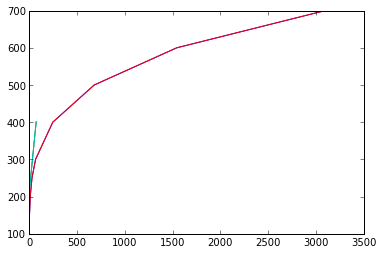# Chapter 16 : Single Component Two Phase Systems¶

### Example 16.1 Page no. 486¶

In :
import math

# Variables
Tc =  972. ;			#[degree C]
T = 273.+Tc ;			#[K]
A = 8.799;
B = 1.615 * 10**4;
C = 0.;
mw = 26.98;

# Calculations
# Use Antoine eqn. to get vapour pressure at 972 degree C
vP = math.exp(A-(B/(C+T))) ;			# vapour pressure at 972 degree C-[mm Hg]
P = vP * 101.325/760 ;			#[kPa]
# Use rate of vapourization(m) by given formula
m = 0.437 * (P * (mw**.5)/(T**0.5)) ;			# Vapourization rate at 972 degree C-[g/(square centimetre * s)]

# Results
print ' Vapourization rate at 972 degree C is %.1e g/(square centimetre)(s).'%m

 Vapourization rate at 972 degree C is 1.3e-04 g/(square centimetre)(s).


### Example 16.3 Page no. 494¶

In :
# Variables
T1 = 110. ;			# Temperature of chlorobenzene - [degree C]
T1F = (9*T1)/(5) + 32 ;			# Temperature of chlorobenzene - [degree F]
P1 = 400. ;			#Pressure of chlorobenzene - [mm of Hg]
P1_psia = P1*14.7/760 ;			#Pressure of chlorobenzene - [psia]
T2 = 205. ;			# Temperature of chlorobenzene - [degree C]
T2F = (9.*T2)/(5) + 32 ;			# Temperature of chlorobenzene - [degree F]
P2 = 5. ;			#Pressure of chlorobenzene - [atm]

# Calculations
P2_psia = P2*14.7 ;			#Pressure of chlorobenzene - [psia]

# Data from steam table
x1 = [.9487,3.72,11.525,29.8,67,247,680,1543,3094];
y1 = [100,150,200,250,300,400,500,600,700];

x2 = [P1_psia,P2_psia];
y2 = [T1F,T2F];
import matplotlib.pyplot as plt

# Results
plt.plot(x1,y1);
plt.plot(x2,y2);
plt.plot(x1,y1);
plt.plot(x2,y2);
plt.show()
vp1 = 150. ;			# vapour pressure of chlorobenzene from cox chart prepared at 245 degree C
vp2 = 700. ;			# vapour pressure of chlorobenzene from cox chart prepared at 359 degree C

print 'Temperature             Estimated vapour pressure of chlorobenzene from cox chart'
print ' 245 degree C            %i psia'%vp1
print ' 359 degree C            %i psia'%vp2Temperature             Estimated vapour pressure of chlorobenzene from cox chart
245 degree C            150 psia
359 degree C            700 psia


### Example 16.4 Page no. 495¶

In :
# Variables
OP_Et = 400. ;			#OSHA PEL of ethyl acetate -[ppm by volume]
OP_Mek = 200. ;			#OSHA PEL of  Methyl ethyl ketone [ppm by volume]
OP_Nba = 1.3 ;			#OSHA PEL of n-butyl acetate [ppm by volume]
vp_Et =   96.9 ;			# Vapour pressure of ethyl acetate obtained from CD-[mm of Hg]
vp_Mek =   94.8 ;			# Vapour pressure of Methyl ethyl ketone obtained from CD-[mm of Hg]
vp_Nba =   20. ;			# Vapour pressure of n-butyl acetate obtained from Perry-[mm of Hg]

# Calculations
Chz_Et = vp_Et/OP_Et ;			#  Combined hazard criterion of ethyl acetate
Chz_Mek = vp_Mek/OP_Mek ;			#  Combined hazard criterion of Methyl ethyl ketone
Chz_Nba = vp_Nba/OP_Nba ;			#  Combined hazard criterion of n-butyl acetate

# Results
print 'Combined hazard criterion of solvents in increasing order are :'
print 'Ethyl acetate :              %.2f'%Chz_Et
print 'Methyl ethyl ketone :        %.2f'%Chz_Mek
print 'n-butyl acetate :            %.2f'%Chz_Nba

Combined hazard criterion of solvents in increasing order are :
Ethyl acetate :              0.24
Methyl ethyl ketone :        0.47
n-butyl acetate :            15.38

In [ ]: